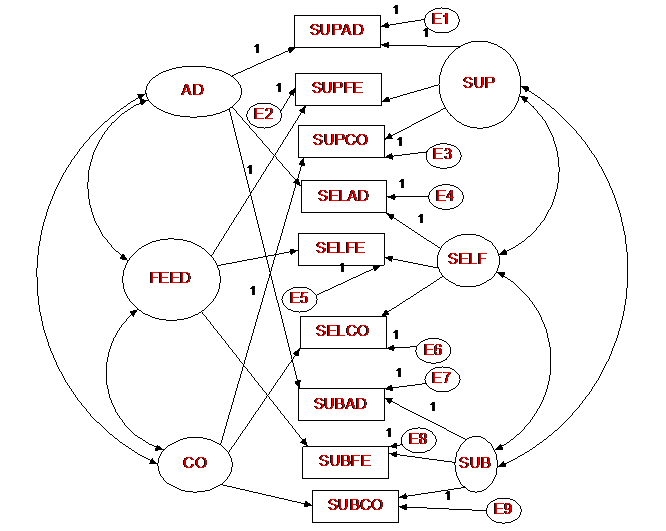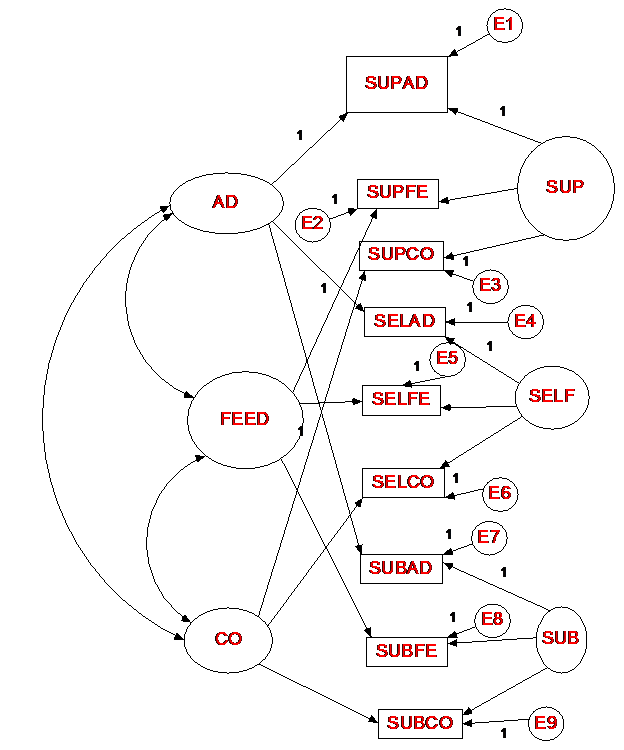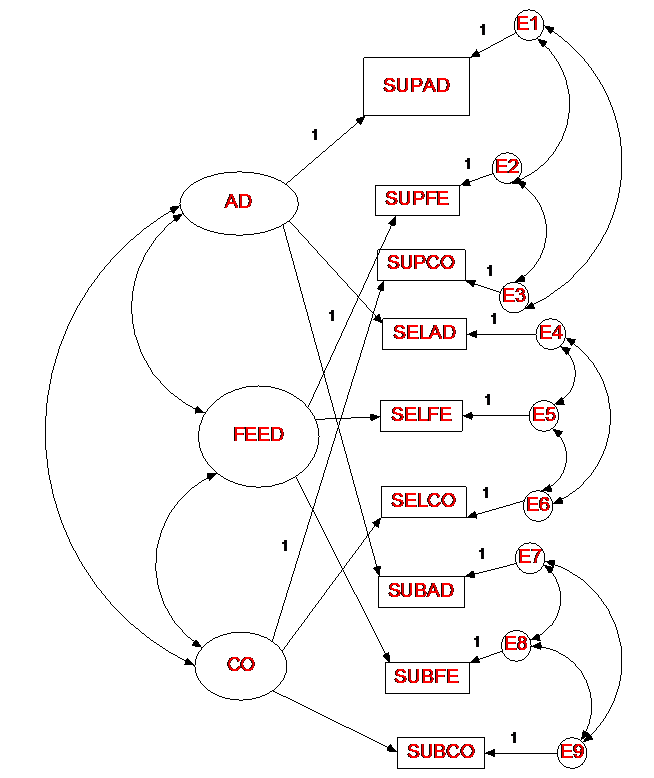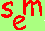David A. Kenny
March 18, 2012
(thanks to Jim Conway)

Page recently revised.

Multitrait Multimethod Matrix

Definitions and Introduction

A set of t traits are each measured by m methods.  The resulting data are tm measures, and the correlation matrix is called a multitrait-multimethod matrix.  The matrix was originally proposed by Donald T. Campbell and Donald Fiske (1959).  The matrix is commonly abbreviated as MTMM.

Types of methods

instrument-based: Guttman, Likert, and Thurstone

occasion-based: times 1, 2, and 3

informant-based: self, supervisor, supervisee

The Matrix

Types of Correlations

homotrait-heteromethod (same-trait, different-method)

heterotrait-homomethod (different-trait, same-method)

heterotrait-heteromethod (different-trait, different method)

Information

1. Convergent validity: measures of the same trait should be strong (Same-trait, different-method correlations are in bold and called the validity diagonal.).

2. Discriminant validity: A measurement method should discriminate between different traits.  Different‑trait, different-method correlations should not by too high, especially relative to same-trait, different-method correlations.

3. Method variance: Variance due to method can be detected by seeing if the different-trait, same‑method correlations are stronger than the different-trait, different-method correlations.

Example

Mount (1984) presented ratings of managers on Administration, Feedback, and Consideration by the managers' supervisors, the managers themselves, and their subordinates (3 traits x 3 methods).

Supervisor           Self                   Subordinate
A         F       C      A       F      C        A        F       C
Supervisor
A       1.00
F        .35    1.00
C       .10    .38    1.00
Self
A       .56    .17    .04    1.00
F       .20    .26    .18    .33    1.00
C       -.01   -.03   .35    .10    .16    1.00
Subordinate
A       .32   .17     .20    .27    .26    -.02   1.00
F       -.03   .07    .28    .01    .17    .14    .26    1.00
A       -.10  .14    .49    .00    .05    .40    .17      .52   1.00

bold correlations: validity diagonal

Currently Infrequently Used Data Analytic Methods for MTMM Data

EFA

ANOVA

Campbell-Fiske Rules of Thumb

Campbell-Fiske approach to MTMM analysis: eyeball the correlations.  Look for:

1. Convergent validity: measures of the same trait should converge or agree.  Same-trait, different-method correlations are in bold ("validity diagonals").

2. Discriminant validity: A measurement method should discriminate between different traits.  Different-trait, different-method correlations should not by too high, especially relative to same-trait, different-method correlations.  If higher, there is method variance.

3. Method variance:  If there were no method variance, the different-trait, same‑method correlations would be the same as the different-trait, different-method correlations.

Limitations: no standard for "good" results, not very precise (e.g., no proportions of trait and method variance).

Standard CFA Estimation

The standard confirmatory factor analysis model of the MTMM is to have each measure load on its trait and method factors.  The traits factors are correlated, as well as the method factors.  Usually, the trait and method factors are assumed to be independent.  There must be at least three traits and methods for this approach to be identified.  The factor loading structure is as follows:

Factor
Trait                   Method
Measures  1        2        3        1        2        3

T1M1         x                           x
T2M1         x                                    x
T3M1         x                                              x
T1M2                   x                x
T2M2                   x                           x
T3M2                   x                                    x
T1M3                            x        x
T2M3                            x                 x
T3M3                            x                           x

where an "x" means that the measure loads on the relevant trait or method factor and no “x” implies a zero loading.

The major advantage of Standard CFA MTMM approach with correlated errors is that the variance of a measure can be orthogonally partitioned into trait, method, and error variance.

Standard CFAIdentification Issues with Standard CFA Model

The standard model requires at least a total 6 trait and method factors with at least 2 trait and 2 method factors.  However, the standard CFA model for the MTMM is not empirically identified for two very important cases:  when the loadings for each factor are exactly equal or when there is no discriminant validity between two or more factors.  Although actual data never exactly satisfy these conditions, they usually approach one of the cases, and so the standard CFA model is typically empirically underidentifiedHeywood cases, impossible values (correlations larger than one and negative  variances), and convergence problems are quite commonly found during estimation.  Thus given these problems, the "standard" model can be problematic.  However, if there are either a large number of traits or methods (5 or more), these estimation problems may be alleviated.

Marsh and Bailey (1991) report that 77% time improper solutions result from the Standard CFA approach.  Very often Heywood cases, there are Heywood cases.  For instance for the example, five of the measures have non-significant error variances.

very strong correlations

uninterruptable results

failure to converge

wild estimates and huge standard errors

Even when this model appears to produce a good solution, trait variance tends to be "pushed" into method factors.

Source of the Problems

Empirical underidentification (despite the fact that fit is almost always excellent!)

Specialized submodels underidentified

Estimation dilemma

While these models have identification issues, it appears that models with a large (5 or more) number of traits, do not have such severe estimation problems.

For the Mount data the fit is quite good, χ²(12) = 9.19, p = .69.  There are no Heywood cases, but several of the trait loadings are weak and one is negative.

Standard CFA with

Uncorrelated Method Factors

This model is identical to the Standard CFA Model, but the method factors are uncorrelated.  Such a model has fewer estimation problems than the Standard CFA Model.   The fit of the model is χ²(15) = 18.73, p = .226. Here is the diagram:Correlated Uniqueness Model

In this model, there are no method factors, but measures that share a common method have correlated errors or uniquenesses.  The error variance-covariance matrix would be as follows:

T1M1   x
T2M1   x      x
T3M1   x      x       x
T1M2                                x
T2M2                                x       x
T3M2                                x       x       x
T1M3                                                                 x
T2M3                                                                 x       x
T3M3                                                                 x       x       x
T1M1  T2M1  T3M1  T1M2  T2M2  T3M2  T1M3  T2M3 T3M3

where an "x" means that a free error variance or covariance and no “x” implies a zero covariance.

For this model to be identified there must be at least two traits and three methods.  This model does not have the difficulties that the standard CFA model has.

Represents traits (trait loadings & trait factor correlations) the same way as standard model and uncorrelated methods model.  The difference is in how method variance is represented: There are no method factors.  Instead, method variance is modeled using uniquenesses (what's left over in a measured variable after trait variance is accounted for).  If there are method effects, the uniquenesses should be correlated.

Identification: at least two traits and three methods.

Marsh & Bailey: proper solutions 98% of the time

almost always converges

usually interpretable results

No real method factors and so method variance difficult to measure

Method does not allow for the decomposition of variance into trait, method and error like the prior two methods.

Strong assumptions

Trait-method and method-method correlations zero

Example

Good Fit: χ²(15) = 18.73, p = .226

Sup A         .758

Sup F                       .385

Sup C                                     .661

Self A          .716

Self F                        .638

Self C                                      .590

Sub A         .441

Sub F                       .244

Sub C                                      .579

by trait: Admin (.638), Feedback (.422), and Consid (.610)

by method: Sup (.601), Self (.648), and Sub (.421)

discriminant validity

trait correlations; rAF = .475, rAC = .020, and rFC = .351.; good discriminant validity

method variance:  average covariance of errors, ignoring sign (assuming correlations were inputted as data)

.177 Supervisor

.088 Self

.252 Subordinate

most method “variance” for the subordinate

Correlated UniquenessMultiplicative or Direct Product Model

Warning:  This model in non-intuitive and difficult to follow.

This model was originally proposed by Campbell & O'Connell who found that method variance was not constant (same value added to every correlation), but rather was proportional to the different-method, different trait correlation.

The model assumes that the correlation between two variables is NOT an additive combination of trait effects and method effects (models described above assume that the correlation a function of the shared trait variance plus the shared method variance).  The multiplicative model assumes that the correlation is a multiplicative function of trait similarity and method similarity.  So, if two methods are completely dissimilar, the correlation the same trait measured by the two methods would be zero.

The correlation between two traits (D and F) with two methods (1 and 2) equals:

rD1,F2 = cD1cF2rDFr12

rD1,D2 = cD1cD2r12

rD1,F1 = cD1cF1rDF

cD1 -- the square root of the communality of measure D1 (the square root of one minus the error variance)

cF2 -- the square root of the communality of measure F2

rDF -- the correlation between traits D and F

r12 -- the overall similarity between methods 1 and 2

Interpretation

Variance decomposed into trait and error variance.

No measure of method variance.  In fact, using a different method dilutes the true relationship between two latent variables, whereas for other MTMM approaches require different methods to obtain the “true” correlation.

One of the advantages of this method is that it estimates a correlation matrix for the methods.  With this matrix, one can determine the similarity of the different methods.  It is possible that the similarity between methods might be one which would mean that the methods that were nominally different were in fact the same.  In essence, the methods would have no discriminant validity.

There have been a few comparisons between the empirical utility of the standard additive and this newer multiplicative model.  The additive model appears to work better.  Nonetheless, the multiplicative model deserves attention.

The method is not often used, perhaps for the following reasons:

1.  Not intuitive.  No proportions of trait and method variance, difficult to interpret.

2.  Difficult to set up.  You do not believe me? See below?

3.  Studies have shown fairly frequent estimation problems.

4.  Fit tends to be worse than for the additive models.

Estimation (not easy to follow; if you do not believe me strongly suggest looking at the figure below).   Assume there are t traits and m methods.  Initially order the measures from 1 to tm, such that method is fastest moving.

a.  Each measure loads on its own factor, denoted as T from 1 to tm.

b.  The loadings for first trait are all fixed to the same value (a in the figure below) and the other loadings are all free.

c.  For each trait, create m standardized latent variables, denoted as K.  As this done for each method there will be a total of tm such K factors.

d.  Fix the correlations between the “same” K factors, i.e., between the same two methods, to be equal across traits.

e.  Draw the following paths form the K to the T factors: For the first m K factor, fix the loadings to 1 for the first set, and then have them load on the other t – 1 sets, but fix the loadings to be the same.  For the last set, just load on the last set.  The K correlations will give the method correlations to establish method similarity.

f.  When done flip the measures and traits such that traits are fastest moving.  This will give the trait correlations that can be used to establish discriminant validity.

For the Mount example, the trait correlations are rAF = .451, rAC = .109, and rFC = .487 and the correlations between methods rSupSel = .510, rSupSub = .273, and rSelSub = .346.  There are also three Heywood cases in the solution.  In terms of model fit χ²(21) = 20.07, p = .96.References

Campbell, D. T., & Fiske, D. W. (1959). Convergent and discriminant validation by the multitrait-multimethod matrix. Psychological Bulletin, 56, 81-105.

Campbell, D. T., & O'Connell, E. J. (1967). Method factors in multitrait-multimethod matrices:  Multiplicative rather than additive? Multivariate Behavioral Research, 2, 409-426.

Kenny, D. A., & Kashy, D. A. (1992). Analysis of multitrait-multimethod matrix by confirmatory factor analysis. Psychological Bulletin, 112, 165-172.

Marsh, H., & Bailey, M. (1991). Confirmatory factor analysis of multitrait-multimethod data: A comparison of alternative models.  Applied Psychological Measurement, 15, 47-70.

Wothke, W. (1984). The estimation of trait and method components in multitrait multimethod measurement. Unpublished Doctoral Dissertation, University of Chicago.Go to the main SEM page.